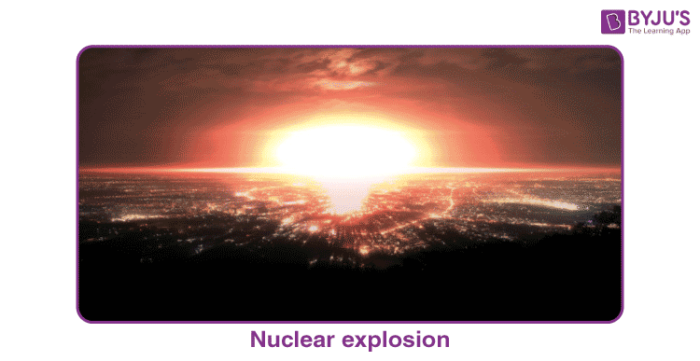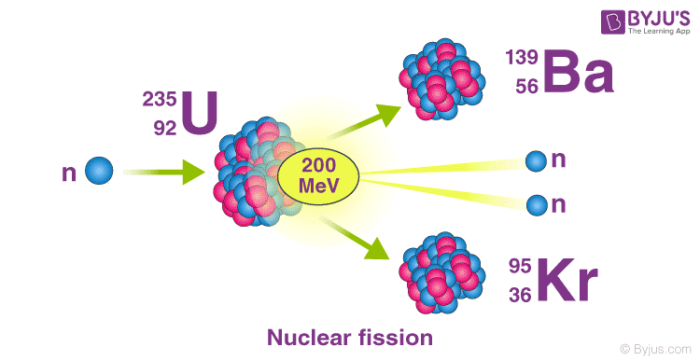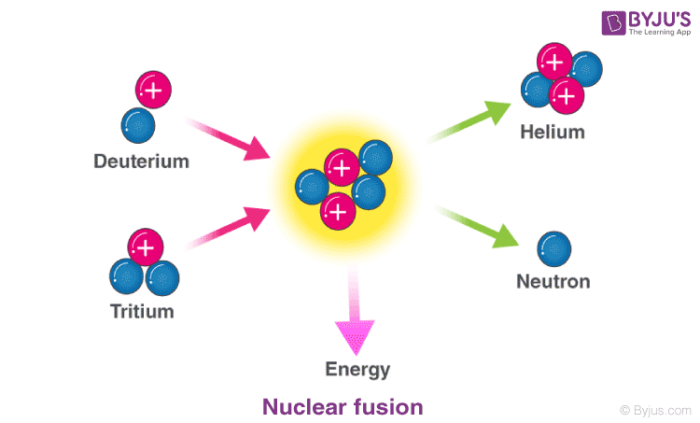Checkout JEE MAINS 2022 Question Paper Analysis : Checkout JEE MAINS 2022 Question Paper Analysis :

# Nuclear Reaction

## What are Nuclear Reactions?

Nuclear reactions are processes in which one or more nuclides are produced from the collisions between two atomic nuclei or one atomic nucleus and a subatomic particle. The nuclides produced from nuclear reactions are different from the reacting nuclei (commonly referred to as the parent nuclei).Two notable types of nuclear reactions are nuclear fission reactions and nuclear fusion reactions. The former involves the absorption of neutrons (or other relatively light particles) by a heavy nucleus, which causes it to split into two (or more) lighter nuclei. Nuclear fusion reactions are the processes in which two relatively light nuclei combine (via a collision) to afford a single, heavier nucleus.

### Processes that are not Considered to be Nuclear Reactions

The term ‘nuclear reaction’ is generally used to refer to the externally induced changes brought on to atomic nuclei. Therefore, the following processes cannot be classified as nuclear reactions:

• Nuclear scattering processes – processes that involve the collision and subsequent separation of atomic nuclei without any notable changes in the nuclear composition. In these processes, only momentum and energy are transferred.
• Nuclear Decay – is a process through which an unstable nucleus emits radiation in order to lose energy.
• Spontaneous fission reactions – nuclear fission reactions that do not require a neutron to proceed and are, therefore, not induced.

These processes are quite similar to nuclear reactions (but are spontaneous rather than induced).

## Why do Nuclear Reactions Release Tremendous Amounts of Energy?

The mass of an atomic nucleus is always less than the sum of the individual masses of each subatomic particle that constitutes it (protons and neutrons). This difference in mass is attributed to nuclear binding energy (often referred to as a mass defect). Nuclear binding energy can be defined as the energy required to hold all the protons and neutrons within the nucleus.

During a nuclear reaction (such as a fission or fusion reaction), the mass accounted for by the nuclear binding energy is released in accordance with the equation e = mc2 (energy = mass times the square of the speed of light).

To simplify, the products formed in nuclear fission and nuclear fusion always have a lower mass than the reactants. This ‘missing’ mass is converted into energy. A single gram of matter can release approximately 90,00,00,00,000 kilojoules of energy.

## Nuclear Fission

Nuclear fission refers to the splitting of an atomic nucleus into two or lighter nuclei. This process can occur through a nuclear reaction or through radioactive decay. Nuclear fission reactions often release a large amount of energy, which is accompanied by the emission of neutrons and gamma rays (photons holding huge amounts of energy, enough to knock electrons out of atoms).Nuclear fission was first discovered by the German chemists Otto Hahn and Fritz Strassmann in the year 1938. The energy produced from fission reactions is converted into electricity in nuclear power plants. This is done by using the heat produced from the nuclear reaction to convert water into steam. The steam is used to rotate turbines in order to generate electricity.

### Examples

An important example of nuclear fission is the splitting of the uranium-235 nucleus when it is bombarded with neutrons. Various products can be formed from this nuclear reaction, as described in the equations below.

• 235U + 1n → 141Ba + 92Kr + 3 1n
• 235U + 1n → 144Xe + 90Sr + 2 1n
• 235U + 1n → 146La + 87Br + 3 1n
• 235U + 1n → 137Te + 97Zr + 2 1n
• 235U + 1n → 137Cs + 96Rb + 3 1n

Another important example of nuclear fission is the splitting of the plutonium-239 nucleus.

## Nuclear Fusion

In nuclear fusion reactions, at least two atomic nuclei combine/fuse into a single nucleus. Subatomic particles such as neutrons or protons are also formed as products in these nuclear reactions.An illustration of the nuclear fusion reaction between deuterium (2H) and tritium (3H) that yields helium (4He) and a neutron (1n) is provided above. Such fusion reactions occur at the core of the sun and other stars. The fusion of deuterium and tritium nuclei is accompanied by a loss of approximately 0.0188 amu of mass (which is completely converted into energy). Approximately 1.69*109 kilojoules of energy are generated for every mole of helium formed.

## Other Important Types of Nuclear Reactions

### Alpha Decay

Nuclei with mass numbers greater than 200 tend to undergo alpha decay – a process in which a 4He nucleus, commonly referred to as an alpha particle (42α) is liberated from the parent nucleus.

The general equation for alpha decay is: AZX → (A-4)(Z-2)X’ + 42α

Where A is the mass number and Z is the atomic number. An example of alpha decay is provided below.

226Ra → 222Rn + 42α

Here, the radium-226 nucleus decays into a radon-222 nucleus, liberating an alpha particle in the process.

### Beta Decay

Beta decay occurs when a neutron is converted into a proton, which is accompanied by the emission of a beta particle (high-energy electron). An example of this type of nuclear reaction is the beta decay of carbon-14 that affords nitrogen-14:

146C → 147N + 0-1β

### Gamma Emission

Gamma emission occurs when an excited nucleus (often produced from the radioactive decay of another nucleus) returns to its ground state, which is accompanied by the emission of a high energy photon.

An example of gamma emission is the de-excitation of the excited thallium-234 nucleus (which is produced from the alpha decay of uranium-238). The equation for this nuclear reaction is:

234Th* → 234Th + 𝜸